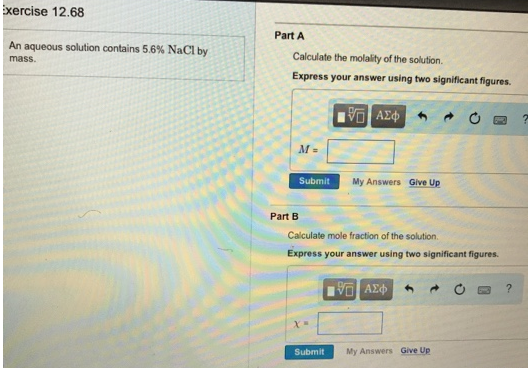# Problem: An aqueous solution contains 56% NaCl by mass.Part ACalculate the molality of the solution. Express your answer using two significant figures. Part B Calculate mole fraction of the solution.Express your answer using two significant figures.

###### FREE Expert Solution
84% (210 ratings)###### Problem Details

An aqueous solution contains 56% NaCl by mass.

Part A

Calculate the molality of the solution.

Part B

Calculate mole fraction of the solution.